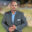Related Tags

linux

# What are relational operators in shell scripting?Kedar Kodgire

## Overview

Operators perform operations on variables/values. The relational operators in Linux are used to determine relations between two variables/values.

Note: The relational operators only work with numeric values. For example, we can use them with (10, 20, "10", "20") and not the strings ("ten", "twenty").

The relational operators are used in the conditionals, such as the if condition in shell scripting. Let's check out the operators along with an example.

### Example

#!/bin/sh

a=9
b=10

if [ $a -eq$b ]
then
echo "[$a -eq$b]: The condition passed as a is equal to b"
else
echo "[$a -eq$b]: The condition failed as a is not equal to b"
fi

if [ $a -ne$b ]
then
echo "[$a -ne$b]: The condition passed as a is not equal to b"
else
echo "[$a -ne$b]: The condition failed as a is equal to b"
fi

if [ $a -gt$b ]
then
echo "[$a -gt$b]: The condition passed as a is greater than b"
else
echo "[$a -gt$b]: The condition failed as a is not greater than b"
fi

if [ $a -lt$b ]
then
echo "[$a -lt$b]: The condition passed as a is less than b"
else
echo "[$a -lt$b]: The condition failed as a is not less than b"
fi

if [ $a -ge$b ]
then
echo "[$a -ge$b]: The condition passed as a is greater than or  equal to b"
else
echo "[$a -ge$b]: The condition failed as a is not greater than or equal to b"
fi

if [ $a -le$b ]
then
echo "[$a -le$b]: The condition passed as a is less than or  equal to b"
else
echo "[$a -le$b]: The condition failed as a is not less than or equal to b"
fi

Note: You can change the values of a and b in the example and try out different use cases.

In the example above, we use the if-else conditions in shell scripting and the relational operators.

## Explanation

### The -eq operator

Lines 6–11: These lines show the use of the -eq operator. The -eq operator validates the values in two variables, and the condition becomes true if they are equal; otherwise, it fails. Then we print the statements accordingly.

### The -ne operator

Lines 13 –18: These lines show the use of the -ne operator. The -ne operator validates the values in two variables, and the condition becomes true if they are not equal; otherwise, it fails. Then we print the statements accordingly.

### The -gt operator

Lines 20–25: These lines show the use of the -gt operator. The -gt operator validates the values in two variables, and the condition becomes true if the first variable is greater than the second; otherwise, it fails. Then we print the statements accordingly.

### The -lt operator

Lines 27 –32: These lines show the use of the -lt operator. The -lt operator validates the values in two variables, and the condition becomes true if the first variable is smaller than the second; otherwise, it fails. Then we print the statements accordingly.

### The -ge operator

Lines 34–39: These lines show the use of the -ge operator. The -ge operator validates the values in two variables, and the condition becomes true if the first variable is greater than or equal to the second; otherwise, it fails. Then we print the statements accordingly.

### The -le operator

Line 41–46: These lines show the use of the -le operator. The -le operator validates the values in two variables, and the condition becomes true if the first variable is less than or equal to the second; otherwise, it fails. Then we print the statements accordingly.

RELATED TAGS

linux

CONTRIBUTORKedar Kodgire
RELATED COURSES

View all Courses

Keep Exploring

Learn in-demand tech skills in half the time